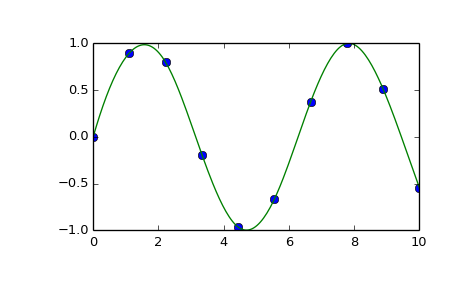# scipy.interpolate.splrep¶

scipy.interpolate.splrep(x, y, w=None, xb=None, xe=None, k=3, task=0, s=None, t=None, full_output=0, per=0, quiet=1)[source]

Find the B-spline representation of 1-D curve.

Given the set of data points (x[i], y[i]) determine a smooth spline approximation of degree k on the interval xb <= x <= xe.

Parameters: x, y : array_like The data points defining a curve y = f(x). w : array_like, optional Strictly positive rank-1 array of weights the same length as x and y. The weights are used in computing the weighted least-squares spline fit. If the errors in the y values have standard-deviation given by the vector d, then w should be 1/d. Default is ones(len(x)). xb, xe : float, optional The interval to fit. If None, these default to x and x[-1] respectively. k : int, optional The order of the spline fit. It is recommended to use cubic splines. Even order splines should be avoided especially with small s values. 1 <= k <= 5 task : {1, 0, -1}, optional If task==0 find t and c for a given smoothing factor, s. If task==1 find t and c for another value of the smoothing factor, s. There must have been a previous call with task=0 or task=1 for the same set of data (t will be stored an used internally) If task=-1 find the weighted least square spline for a given set of knots, t. These should be interior knots as knots on the ends will be added automatically. s : float, optional A smoothing condition. The amount of smoothness is determined by satisfying the conditions: sum((w * (y - g))**2,axis=0) <= s where g(x) is the smoothed interpolation of (x,y). The user can use s to control the tradeoff between closeness and smoothness of fit. Larger s means more smoothing while smaller values of s indicate less smoothing. Recommended values of s depend on the weights, w. If the weights represent the inverse of the standard-deviation of y, then a good s value should be found in the range (m-sqrt(2*m),m+sqrt(2*m)) where m is the number of datapoints in x, y, and w. default : s=m-sqrt(2*m) if weights are supplied. s = 0.0 (interpolating) if no weights are supplied. t : array_like, optional The knots needed for task=-1. If given then task is automatically set to -1. full_output : bool, optional If non-zero, then return optional outputs. per : bool, optional If non-zero, data points are considered periodic with period x[m-1] - x and a smooth periodic spline approximation is returned. Values of y[m-1] and w[m-1] are not used. quiet : bool, optional Non-zero to suppress messages. This parameter is deprecated; use standard Python warning filters instead. tck : tuple (t,c,k) a tuple containing the vector of knots, the B-spline coefficients, and the degree of the spline. fp : array, optional The weighted sum of squared residuals of the spline approximation. ier : int, optional An integer flag about splrep success. Success is indicated if ier<=0. If ier in [1,2,3] an error occurred but was not raised. Otherwise an error is raised. msg : str, optional A message corresponding to the integer flag, ier.

See also

Notes

See splev for evaluation of the spline and its derivatives. Uses the FORTRAN routine curfit from FITPACK.

If provided, knots t must satisfy the Schoenberg-Whitney conditions, i.e., there must be a subset of data points x[j] such that t[j] < x[j] < t[j+k+1], for j=0, 1,...,n-k-2.

References

Based on algorithms described in [R66], [R67], [R68], and [R69]:

 [R66] (1, 2) P. Dierckx, “An algorithm for smoothing, differentiation and integration of experimental data using spline functions”, J.Comp.Appl.Maths 1 (1975) 165-184.
 [R67] (1, 2) P. Dierckx, “A fast algorithm for smoothing data on a rectangular grid while using spline functions”, SIAM J.Numer.Anal. 19 (1982) 1286-1304.
 [R68] (1, 2) P. Dierckx, “An improved algorithm for curve fitting with spline functions”, report tw54, Dept. Computer Science,K.U. Leuven, 1981.
 [R69] (1, 2) P. Dierckx, “Curve and surface fitting with splines”, Monographs on Numerical Analysis, Oxford University Press, 1993.

Examples

>>> import matplotlib.pyplot as plt
>>> from scipy.interpolate import splev, splrep
>>> x = np.linspace(0, 10, 10)
>>> y = np.sin(x)
>>> tck = splrep(x, y)
>>> x2 = np.linspace(0, 10, 200)
>>> y2 = splev(x2, tck)
>>> plt.plot(x, y, 'o', x2, y2)
>>> plt.show()#### Previous topic

scipy.interpolate.LSQUnivariateSpline.set_smoothing_factor

#### Next topic

scipy.interpolate.splprep